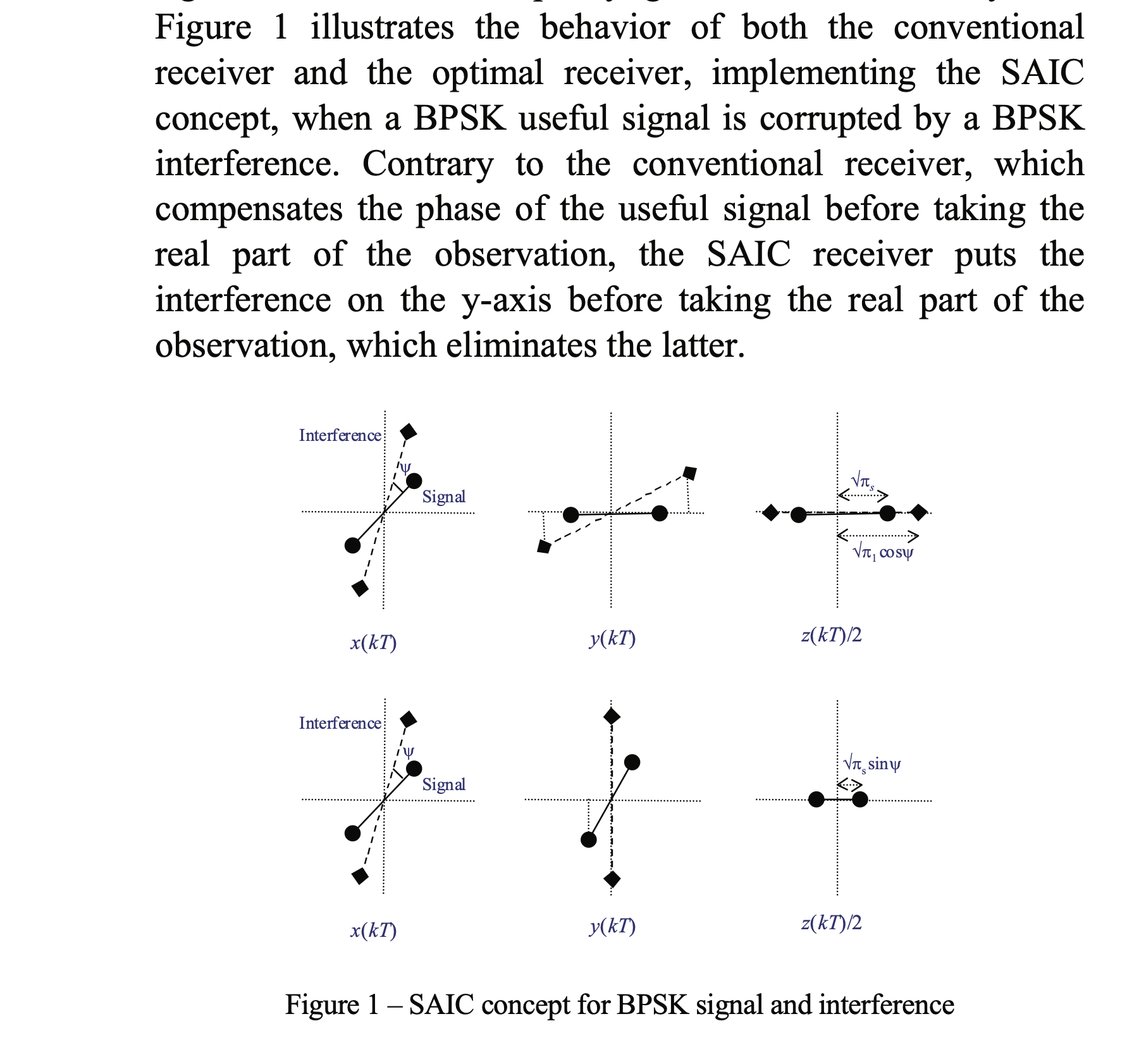Posted on 15 January 2023

Here’s some interesting papers and webpages that I have hanging around in open browser tabs. Better to have them here than languishing in browser tabs/history/bookmarks!

## signal processing stuff #

### spicy signal processing: beyond circularity and linearity #

• normal filters add up multiple copies of the same signal, but time-offset
• array processing adds up multiple copies of the same signal, but space-offset
• FRESH (FREquency SHift) filters add up multiple copies of the same signal, but frequency-offset
• this is useful because many signals (like communication/radar RF signals) have redundancy/correlation in their frequency domain (a property called cyclostationarity)
• “Noncircularity exploitation in Signal Processing Overview and Application to Radar” by F. Barbaresco, Pascal Chevalier; about widely linear processing/filtering/estimation

• a lot of time it’s justified to assume that complex-valued signals through complex-valued systems behave the same as real valued signals and systems (and using the same sort of filters / estimators you’d use for real-valued everythings)
• pretending that complex signals work just like real signals depends on an assumption called “second-order circularity”
• second-order circularity doesn’t always hold!
• for instance if the signal (prior to passing through the channel) only takes a real value (like -1 or 1, like with a BPSK), then there’s a fundamental asymmetry between the inphase and quadrature channels, and that violates the second-order circularity assumption.
• note: a symmetric QAM signal (modulated with random data, as always) is itself not circularly symmetric (add a phase offset and the little square lattice gets tilted) but it is second-order circular
• if second-order circularity doesn’t hold and you process the received signal in a way that can’t tease apart the asymmetry then you are leaving signal on the table.
• in the case where the modulated signal is only real-valued (or can be transformed to be only real-valued) that special signal structure morally lets you get a sort of processing gain because you know that any variation in the complex axis is noise/interference/etc:• a linear filter looks like $$y = h\cdot x$$ ($$y$$ output, $$h$$ coefficients, $$x$$ input), the widely-linear model looks like $$y = g \cdot x + h \cdot x^*$$ ($$y$$ output, $$h$$ and $$g$$ coefficients, $$x$$ input, and $$x^*$$ the complex conjugate of $$x$$) – so it’s linear in both $$x$$ and its complex conjugate $$x^*$$
• as i understand it, this lets the system do stuff like “take only the real part of the signal” (because the noise all lives in the imaginary axis) but in a principled way
• “Widely Linear Estimation with Complex Data”, by Bernard Picinbono, Pascal Chevalier, also about widely linear processing

• “Receivers with widely linear processing for frequency-selective channels” by H. Gerstacker; R. Schober; A. Lampe: more about widely linear processing

• Widely linear filtering isn’t new: “Conjugate linear filtering” by W. Brown; R. Crane is from 1969!

• “Enhanced widely linear filtering to make quasi-rectilinear signals almost equivalent to rectilinear ones for SAIC/MAIC” by Pascal Chevalier, Rémi Chauvat, Jean-Pierre Delmas

• we saw earlier that if a signal (as transmitted) has a special form and only lives in the reals (like BPSK or a PAM), this allows for a form of processing gain at the receiver
• even more interestingly, this allows for signal separation / interference cancellation (if both the desired and interfering signal are of this form): the receiver can adjust the phase of the received signal until the desired signal lives only on the reals (this is a linear operation), and trash the imaginary component of the signal altogether
• the real-world realization is more complex since there are two channels (desired signal channel, interferer signal channel) that need to be taken into account, but this actually works: it’s called “single antenna interference cancellation” (SAIC)
• the titles of those papers implies that this is deployed for GSM networks, which notably uses GMSK, which is definitely not BPSK nor a PAM
• however, it turns out we can use this “single antenna interference cancellation” for certain modulations that aren’t BPSK or a PAM, with an additional step: the infamous “derotation”, which converts an MSK into BPSK, and converts GMSK into an almost-BPSK (“almost” because of the second Laurent pulse)
• this paper goes well beyond the standard SAIC; looking into both widely-linear filtering and FRESH filtering, in order to exploit the spectral structure of the signal of interest
• two books i found that might be useful later